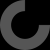Hey! This thing is still a Work in Progress. Files, instructions, and other stuff might change!

# Occlupanidae

by grokbeer Feb 5, 2012

### Thing Apps Enabled

I came here searching for a giant bread tag and I was not disappointed. Truly a momentous occasion. Thank you for your contribution.

This is awesome! Have you proceeded with your extending the script to create various species? If you want some help, I'd be interested.

BTW, I went through the script and added tiny offsets so that the subtracted volumes faces don't coincide with the faces of the objects being subtracted from, which lets it render more cleanly. It's not enough to call it a 'derivative' so I'll just post examples of the changes. Basically, I subtra
cted a tiny bit from the z axis on all of the subtracted shapes, and made then twice that much taller. And the trim corners had to start slightly outside the 'body' for the same reason.

t=0.1; t2=0.2; // used for offsets to avoid overlapping faces

``````    // lesser distal palp notch
translate( [ 0 - ``````

( palp_notch_radius - palp_notch_depth ), body_y - palp_notch_radius, -t ] ) {
cylinder( r = palp_notch_radius, h = body_z+t2 );
}

``````    // oral proximal notch
translate( [ body_x/2 - oral_proximal_notch_x/2, -t, -t ] ) {
linear_extrude( height = body_z+t2 ) {
polygon( points = [ [0,0], [``````

oral_proximal_notch_x/2,oral_proximal_notch_y], [oral_proximal_notch_x,0] ], paths = [ [0,1,2] ] );
}
}

``````    // trim corner (sinister proximal)
translate([-t,-t,-t]) linear_extrude( height = body_z+t2 ) {
polygon( points = [ [0,0], [0,corner_trim], [corner_trim,0] ], paths = [ [0,1,2] ] );

}

// trim corner (dexter proximal)
translate([t,-t,-t]) linear_extrude( height = body_z+t2 ) {
polygon( points = [ [body_x-corner_trim,0``````

], [body_x,corner_trim], [body_x,0] ], paths = [ [0,1,2] ] );
}

``````    // trim corner (sinster distal)
translate([0,0,-t]) linear_extrude( height = body_z+t2 ) {
polygon( points = [ [0,t+body_y-corner_trim], [0,body_y+t], [corner_trim,body_y+t] ], paths = [ [0,1,2] ] );
}

// trim corner (dex``````

ter distal)
translate([0,0,-t]) linear_extrude( height = body_z+t2 ) {
polygon( points = [ [t+body_x-corner_trim,body_y+t], [body_x+t,body_y+t], [body_x+t,t+body_y-corner_trim] ], paths = [ [0,1,2] ] );
}

module palp( body_z, palp_radius, palp_offset_x ) {
difference() {
cylinder( body_z